﻿ The Presentation of Financial Crisis Forecast Pattern (Evidence from Tehran Stock Exchange) Scientific & Academic Publishing: The article detailed informationInternational Journal of Finance and Accounting

p-ISSN: 2168-4812    e-ISSN: 2168-4820

2012;  1(6): 142-147

doi: 10.5923/j.ijfa.20120106.02

### The Presentation of Financial Crisis Forecast Pattern (Evidence from Tehran Stock Exchange)

1Department, Management & Social Science Faculty, Islamic Azad University, Tehran North Branch, Tehran, Iran

2Master in Accounting, Islamic Azad University, Tehran North Branch, Tehran, Iran

Correspondence to: Mohammad Hassani , Department, Management & Social Science Faculty, Islamic Azad University, Tehran North Branch, Tehran, Iran.
 Email: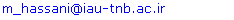Abstract

The purpose of this study is presenting a model for forecasting financial crisis in Tehran Stock Exchange listed companies. To do this, productive firms that had accepted in Tehran Stock Exchange between 2002 and 2009, were selected as the study sample. First the independent variables were obtained based on financial ratios and then based on Article 141 of the Commerce Law, the insolvent and solvent firms were separated. Next, based on these two groups of firms, the presence or absence of meaningful difference between independent variables (financial ratios) of this two groups samples were tested. The results obtained from this test contributed to the extraction of a proper model of forecast using logistic regression. The results indicated that the variables of debt to the equity ratio, net profit to net sales ratio & working capital to assets ratio were identified as independent variables in the final model. In addition, the results indicated that using the test data, the forecast strength of the model is 81.49%, its degree of sensitivity is 96.12% and its degree of identification is 67.48%.

Keywords: Bankruptcy, Financial Ratios, Logistic Regression

Cite this paper: Mohammad Hassani , Nasim Parsadmehr , "The Presentation of Financial Crisis Forecast Pattern (Evidence from Tehran Stock Exchange)", International Journal of Finance and Accounting , Vol. 1 No. 6, 2012, pp. 142-147. doi: 10.5923/j.ijfa.20120106.02.

### 1. Introduction

Nowadays the rapid developments of technology and vast environmental changes have accelerated economy and the ever-increasing competition among institutions has limited gains and increased bankruptcy possibility. Owners, managers, investors, commercial partners and creditors are interested in evaluation of the financial status of a company as much as governmental institutions do. Thus, financial decision making is more strategic now than before. In making financial decisions on any institution, suitable scientific and real indices for the respective institution are needed. One of the suitable indices serving this purpose is correct evaluation of the probability of firm bankruptcy. Financial ratios are among the financial analysis tools and researchers have combined these ratios to devise multivariate models for predicting bankruptcy. Altman, Ohlson, Springate, Wallace, etc. used financial data for predicting bankruptcy of companies.[3, 8, 12, 13].
Bankruptcy prediction models are among the techniques and tools for predicting future status of companies which can estimate the bankruptcy probability by compounding a set of financial ratios. Ability to predict financial and commercial bankruptcy is important both from the viewpoint of private investors and the social viewpoint as it shows incorrect allocation of resources. Having a portent of bankruptcy, the management and the investors can take preventive measures and identify desirable investment opportunities from undesirable ones. This research has attempted to devise a model for predicting financial crisis and bankruptcy probability in sample companies and test the ability of this model in predicting financial crisis.

### 2. Review of literature

Several studies have been done on this subject in Iran and throughout the world as follow:
Abzari et al in their paper titled “Identification of Financial Crisis in Companies on the Basis of Indices” tried to assess the ability of financial ratios in identification of solvent and insolvent companies. They used binary regression model (Logit-Probit) for this purpose. Their model was fitted using the data on two groups of companies listed in Tehran Stock Exchange (regional Khorasan Stock Exchange). The first group comprised of 20 companies that were not in financial crisis and the second group included 20 companies listed in the unofficial stock exchange. Their model included four financial ratios and one economic ratio. The model was dismantled to its components and then underwent regression of financial status of the companies (dependent variable). The statistical results of this research showed incompatibility with other multivariate models in other researches. In single-variable regressions, three independent variables were significant.
Ghadiri Moghaddam et al in their paper titled “To Study the Ability of Bankruptcy Prediction Models of Altman and Ohlson in Predicting Bankruptcy of Stock Exchange Listed Companies” aimed at first, comparing bankruptcy prediction models of Altman and Ohlson in Iranian environment and then, present a suitable statistical model for predicting companies’ bankruptcy, in one, two and three years before the financial crisis occurrence as exact as possible in order to use the model for investigating the financial status of companies and their continuation and improve the quality of decisions to be made by shareholders and beneficiaries.
In “Prediction of Financial Crisis of Companies Using a Model Based on Linear Discriminate Function”, Pour Heidari et al studied important variables in predicting financial crisis and bankruptcy in companies and identified the most important financial variables serving financial crisis prediction. Having identified the most important bankruptcy prediction variables, they presented a model for predicting financial crisis and bankruptcy of companies and then tested the prediction power of their model. They used linear discriminate function to identify the most important variables for predicting financial crisis and bankruptcy of companies and devised a 9 variable model. The ratios used by them included earnings before interest and tax to assets ratio, accumulated income to assets ratio, working capital to assets ratio, equities to debts ratio, earnings before interest and tax to sale ratio, current assets to current debts ratio, net profit to sale ratio, debts to assets ratio and company’s size. The prediction ability of the model was tested using the data related to companies in and out of financial crisis. Based on the results they showed that using their model one can predict financial crisis up to five years earlier with relatively acceptable precision.
Adnan Aziz compared different models for predicting bankruptcy one year prior to bankruptcy and compared the results of 46 researches, 43 papers, one technical report and two inquiry-answer papers in 89 companies and concluded that 60% of the studies used financial ratios, 7% used cash currents data and the other 33% used a combination of financial ratios and other variables such as microeconomic and macroeconomic variables and special industrial and corporate variables as descriptive variables that confirm the validity of company’s accounts information.
Springate continued Altman’s studies and devised a model using working capital to total assets ratio, earnings before interest and tax to total assets ratio, pre-tax profit to current debts ratio and sale to total assets ratio, as independent variables, which enjoyed a precision percentage of 92.5%.
Andrew in his research compared discriminate analysis method with Logit method and concluded that prediction using logit method is more comprehensive and effective. He commented that logit technique is better than discriminate analysis method.
Ohlson was the first person who used logistic regression model for predicting bankruptcy. His sample including 105 insolvent and 2058 solvent companies made his study the most comprehensive one done to the time and his devised model could predict bankruptcy probability of companies one to three years earlier with precision of 85.1%, 87.6% and 82.6% respectively and total debts to total assets ratio and net profit to total assets ratio were the best discriminate ratios in his model.
Dimitras et al covered all aspects of financial crisis literature to improve commercial crisis prediction. They studied 158 papers and classified them on the basis of country, industry, period, financial ratios and models or methods used. Although many solutions were offered for coping with limitations of discriminate analysis method in the 1980s, but their findings showed that discriminate analysis technique was the most widespread system in financial crisis studies.
Ooghe & Balcaen predicted financial crises using four classic statistical methods (univariate analysis, risk index models, multivariate discriminate analysis and conditional probability models). They showed that the four methods can be used to predict financial crisis, but conditional probability models yield better results in comparison with other methods.
Wallace designed a model using neural networks method with the help of key financial ratios that reported as the best ratios. The ratios used by him included working capital to total assets ratio, cash flows to total assets ratio, net profit to total assets ratio, total debts to total assets ratio, current assets to current debts ratio and accelerated assets to current debts ratio. Total precision percentage of Wallace’s model was 94% and he studied 65 different financial ratios reported in the literature.
Sprengers used two techniques of discriminate analysis and regression analysis to distinguish insolvent and solvent companies. The sample included 122 companies active in the stock exchange of the Netherlands during 1945-1999. The researcher concluded that the prediction precision of discriminate analysis was more than regression model.
Min and Lee used learning machine technique for predicting financial crisis. Also, the researchers compared their results with those out of multivariate discriminate analysis, logistic regression analysis and artificial neural networks methods. Using 11 financial ratios as independent variables the researchers inferred that learning machine technique yields better results in comparison with other methods. Furthermore, prediction truth percentage for the control sample was between71% to 83%.

### 3. Methodology

With regard to objective, this research is applied and in terms of methodology it is descriptive (semi-experimental) and correlation type. In this research, by using the logistic regression technique, the best model for predicting bankruptcy in Tehran Stock Exchange listed companies was fitted. The major steps for executing this study were as follows:
- Calculation of financial ratios or required parameters as independent variables in the tested model and calculation of values of the model.
- Discrimination of two samples of solvent and insolvent companies using article 141 of Commercial Code with the help of simple Tobin's q.
- Analysis the truth of discrimination of two solvent and insolvent samples using F test for comparing the mean of independent variables of the two samples.
- Fitting statistical model on the basis of the extracted data.
- Selecting suitable pattern with less error criteria.
To be added, the financial ratios used in this research were extracted based on the conditions of the companies listed in Tehran Stock Exchange. Also, the prediction pattern of this research was presented with due regard to the significant differences of financial ratios of the two sample groups.

### 4. Population, Sampling Method and Sample Size

The research population included the companies listed in Tehran Stock Exchange during 2002 to 2009. The information of solvent and insolvent companies was needed so the companies were classified into two solvent and insolvent groups using the presupposition of article 141 of Commercial Code saying that if a given company is subject to article 141 for three consecutive years it is insolvent, otherwise it is solvent. Simple Tobin’s q was a suitable criterion for selecting successful companies.
Omitting sampling method was used in this research according to the following criteria:
1. Being productive company
2. Being listed before the research time period
3. Fiscal years ending on 20th of March (Iranian fiscal year) and lack of modification of fiscal year during the term of the research
4. Lack of transactional break during the research period

### 5. Data Analysis

#### 5.1. Calculation of Financial Ratios and/or Parameters Needed as Independent Variables of the Tested Model and Discrimination of Solvent and Insolvent Companies

At first, variables like cash, accounts receivable, current assets, total assets, current debts, total debt, working capital, profit and loss, equities, sale, gross profit, net profit and financial expenses were extracted and then leverage ratios, profitability ratios, activity ratios and liquidity ratios were calculated as follow:
* Leverage ratios:
- X1: Debt to Asset Ratio
- X2: Debt to Equity Ratio
- X3: Financial Expenses Coverage Ratio
* Profitability ratios:
- X4: Net Profit to Asset (ROA)
- X5: Net Profit to Net Sale (ROS)
- X6: Gross Profit to Net Sale
- X7: Net Profit to Equity (ROE)
* Activity ratios:
- X8: Accounts Receivable to Net Sale
- X9: Sale to Asset
* Liquidity ratios:
- X10: Current Ratio (Current Asset to Current Debt)
- X11: Quick Ratio (Quick Asset to Current Debt)
- X12: Working Capital to Asset
- X13: Cash to Asset
- X14: Cash to Current Debt
The companies under study were categorized into solvent and insolvent companies by virtue of article 141 of Commercial Code and simple Tobin’s q, say, if a company was subject to article 141 of Commercial Code for three consecutive years it was held to be insolvent, otherwise, it was solvent. Simple Tobin’s q was used to selected successful companies.

#### 5.2. Comparing Means of Independent Variables of Solvent and Insolvent Samples Using F Test

 Table 1. The results of ANOVA test for different ratios
 Variable Symbol MeanError Squares F Significant Level Debt to Asset Ratio X1 008/0 5/110 000/0 Debt to Equity Ratio X2 971/6 8/266 000/0 Financial Expense Ratio X3 149/20 3/175 000/0 Net Profit to Asset Ratio X4 010/0 557/0 458/0 Net Profit to Net Sale Ratio X5 011/0 520/12 001/0 Gross profit to net sale ratio X6 017/0 368/0 546/0 Net Profit to Equity Ratio X7 050/0 399/1 241/0 Accounts Receivable to Net Sale Ratio X8 059/0 112/0 739/1 Sale to Asset Ratio X9 607/0 562/2 114/0 Current Ratio X10 218/0 5/933 000/0 Quick Ratio X11 006/0 513/0 476/0 Working Capital to Asset Ratio X12 042/0 08/30 000/0 Cash to Asset Ratio X13 003/0 469/0 496/0 Cash to Current Debt Ratio X14 006/0 368/2 128/0
F test was used for comparing the mean of independent variables in solvent and insolvent samples. The hypotheses in the test were as follows:
H0: There is no significant difference between the mean of independent variables of solvent and insolvent samples.
H1: There is significant difference between the mean of independent variables of solvent and insolvent samples.
Accordingly,14independent variables were classified into two groups and the mean of variables were calculated one by one. The results of model fitting are mentioned in table 1:
Based on the results of table 1, significant difference among the means of debt to asset ratio, debt to equity ratio, gross profit to financial expenses ratio, net profit to net sale ratio, current ratio, working capital to asset ratio of solvent and insolvent groups were confirmed and significant difference among the means of other ratios were rejected.

#### 5.3. Fitting a Statistical Model on the Basis of Extracted Data

Since, part of the objective of this research was fitting a model using logistic regression method, the model shoud be evaluated in terms of the significance of coefficients and goodness of fit after estimation as the devised model wouldn’t be valid unless the model enjoyed the characteristics of a good fit. It is noticeable that logistic model, due to the binary nature of its dependant variable, is different from normal regression and the tests used for it are completely different from normal ones. Thus, we studied these cases in logistic model and estimated the model and evaluated it.
In order to fit the final research model, first, we estimated the function and the coefficients of each variable. Knowing these coefficients, we put the information of each company in the model and calculated Ŷi of each company. The value was put in the following equation and the quantity of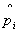was calculated.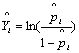Knowingof each company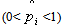, we could predict the bankruptcy probability of each company.
Using the data collected for the variables named in the previous section, we calculated the values for 73 companies listed in Tehran Stock Exchange active during 2002 to 2009 in order to devise an optimal model.
The results of the model fitting with 6 independent variables extracted on the basis of the difference of means are mentioned in the below table:
 Table 2. Results of logistic regression estimation
 Independent variable Coefficient Wald statistic Expected coefficient P-value Constant 5.38 0961 -- 0.327 X1 -3.06 0540 0.047 0.462 X2 -0.18 2.97 -1.2 0.023 X3 -0.009 0.036 0.992 0.850 X5 4.341 3.85 0.835 0.020 X10 -4.580 1.48 0.010 0.223 X12 9.620 2.38 1.50 0.040
Wald statistic was used to evaluate the significance level of logistic regression function. To be added, like the study of the significance level of linear regression function, here the null hypothesis was that the variable had no effect on the dependent variable which was tested using the respective statistics. As you see in the above table significance level of Wald statistic for X2, X5 and X12 is less than 0.05. Thus, the null hypothesis for the named variables is rejected and the coefficients are significant.

#### 5.4. Selecting Suitable Model Using Logistic Regression for Model Fitting

Inter method was used for fitting logistic regression and all the independent variables were entered in the model simultaneously. In the fitted models, the significance level of coefficients was tested using Wald statistic, total significance of regression was tested using LR statistic (P<0.05) and lack of linearity of variables and lack of estimation error were studied. Finally, after testing different compositions of variables and removing unhelpful variables, we identified the following variables as independent variables in the final model:
X2: debt to equity ratio
X5: net profit to net sale ratio
X12: working capital to asset ratio
The results of estimation are entered in table 3:
 Table 3. Significant model variables
 Parameter Values P-value C 4.045 0.0213 X2 -1.341 0.005 X5 0.654 0.020 X12 2.002 0.000
The overall form of the logit function is as follows: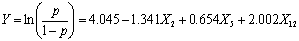Next, we will discuss the significance level of coefficients and model and also will interpret coefficients.
##### 5.4.1. Significance of Coefficients
As mentioned in the previous section, Wald statistic was used to measure the significance of the coefficients of logit regression function variables. Like the null hypothesis in the analysis of the coefficients significance of linear regression function, here the null hypothesis is that the respective variable had no effect on the dependent variable. Table 3 shows that Wald statistic for the three variables is less than 0.05 meaning that the null hypothesis is rejected for all the coefficients; therefore, the coefficients were significant.
##### 5.4.2. Model Fitting Goodness and Significance
Having estimated the coefficients of model independent variables and their significance, we studied the total significance of the regression model and the goodness of its fitness. As mentioned in the previous section, several statistics were used to study the goodness of logit regression model:
√ LR statistic which is similar to F statistic in linear regression model that has X2 distribution (k=3, k is the number of model independent variables). The total significance of the regression model is calculated according to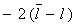formula which value in our model is 93.431.
√ The probability of LR statistic which value is 0.000 (<0.05) shows that the null hypothesis (all the coefficients of independent variables are zero) is rejected at level of significance of 0.95; therefore, the regression is significant.
 Table 4. Statistics related to model goodness of fit
 Model Model likelihood criteria LR ratio test -2Loglikelihood X2 Level of significance Model only with fixed coefficient 109.65 Final model 93.431 45.601 0.000
√ Mc Fadden R-square statistic which is similar to R2 statistic in linear regression is 0.612 in our model. To be added, the value is acceptable according to similar researches for logit model.
 Table 5. Macfadden statistic of the model
 √ Cox and Snell statistics √ 0.501 √ Macfadden statistic √ 0.612
##### 5.4.3. Interpretation of Coefficients
As already mentioned, change of L variable (chance ratio logarithm) shows one unit of change in x variable for all the coefficients.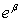shows the effect of one unit of change in independent variable x on chance ratio, i.e. p/1-p (provided that other variables are fixed) (Whitehead J., 2004). Thus, the estimated coefficients can be interpreted as follows:
1. Debt to equity ratio: If other conditions are fixed, this coefficient shows that if debt to equity ratio increases one unit, the logarithm of chance ratio decreases 1.341 units on average in favor of solvency.
2. Net profit to net sale ratio: If other conditions are fixed, this coefficient shows that if net profit to net sale ratio increases one unit, the logarithm of chance ratio increases 0.654 units on average in favor of solvency.
3. Working capital to asset ratio: If other conditions are fixed, this coefficient shows that if working capital to asset ratio increases one unit, the logarithm of chance ratio increases 2.002 units on average in favor of solvency.

#### 5.5. Studying the Model Prediction Power Using Experimental Data

The results of studying the model prediction power are entered in table 6.
 Table 6. Model prediction power
 Observations Prediction 0 1 Truth Percentage 0 13 6 67.48 1 2 52 96.12 Total Percentage 20.54 79.46 81.49
As table 6 shows, the fitted model correctly classified 13 y=0 observations and 52 y=1 observations.
In statistics literature table 6 is called classification table. Y=1 observations which are correctly predicted are called sensitivity coefficient of the model and Y=0 observations which are correctly predicted are called specificity coefficient of the model.
Sensitivity and specificity coefficients of the model are entered as percentage in table 6. All in all, it can be said that the fitted model correctly predicted 96.12% of all Y=1 observations and 67.48% of all Y=0 observations. In other words, the sensitivity of the model is 96.12% and its specificity is 67.48%.
With regard to table 6, type one error (when an insolvent company is mistakenly put in solvent companies group) is 3.88% (1-sensitivity coefficient) and type two error (when a solvent company is mistakenly put in insolvent companies group) is 32.52% (1-specificity coefficient).

### 6. Conclusions and Suggestions

Financial bankruptcy of companies is one of the important issues in financial decision making scope that entails micro and macro consequences in the societies for which prediction noticeable tools and models different in terms of methodology or variables are presented at international level. This paper has presented a logistic regression based model. The results show that there are significant differences among the means of some of financial ratios in two solvent and insolvent groups.
The model presented in this paper can predict financial crisis of companies and based on it, required strategies for control revisions in companies can be used. By controlling the company and adopted required strategies, one can prevent financial crisis and accordingly bankruptcy.

### References

  Abzari, M., et al., 2007, “Identification of Financial Crisis in Companies based on Indices”, The Fifth International Management Conference.  Adnan Aziz, M. and Homayon A. Dar, 2006, “Predicting Corporate Bankruptcy: Where We Stand?”, Corporate Governance Journal, Vol.6, No.1, PP. 18-33.  Altman E.I. , 1968, “Financial ratios, discriminant analysis and the prediction of corporate bankruptcy”, Journal of Finance, Vol. 23, No. 4, PP. 589-609  Dimitras A., Zanakis S., Zopudinis C., 1996,“A survey of business failures with an emphasis on failure prediction methods and industrial applications”, European Journal of Operational Research, Vol. 90, No.3, PP. 487-513.  Ghadiri Moghaddam, A., et al., 2009, “To Study the Capabilities of Altman and Ohlson Bankruptcy Prediction Models in Predicting Bankruptcy of the Companies Listed in Stock Exchange”, Danesh & Toseeh Journal, Issue 28.  Lo, Andrew W, 1986,“Logit versus Discriminant Analysis, A specification test and application to corporate bankruptcy”, University of Pennsylvania, Journal of Econometrics, Volume 31, Issue 2, PP.151-178.  Min, J. H. and Lee, Y. C., 2006,“Bankruptcy Prediction Using Support Vector Machine with Optimal Choice of Kernel Function Parameters”, Available Online.  Ohlson, James A., 1980, “Financial ratios and the probabilistic prediction of bankruptcy”, Journal of Accounting Research, Vol. 18, No. 1, PP. 109-131.  Ooghe, H. and Balcaen, S., 2004, “Alternative Methodologies in Studies on Business Failure: Do They Produce Better Results Than the Classical Statistical Methods?”, Working Paper, Department of Accountancy and Corporate Finance, Ghent University, Belgium.  Pourheidari, O.; Koupaei, H.M., 2010, “Prediction of Financial Crisis of Companies using Linear Discriminate Function Based Model”.  Sprengers, M. A., 2005,“Bankruptcy Prediction by Classification and Regression Trees”, Bachelor Thesis Informatics & Economics, Faculty of Economics, Erasmus University Rotterdam.  Springate, Gord, L.V., 1978, “Predicting the possibility of failure in a Canadian firm”, Unpublished MBA Research Project, Simon Fraser University.  Wallace Wanda A., 2004, “Risk Assessment by Internal Auditors Using Past Research on Bankruptcy Applying Bankruptcy Models”.